Hussain Arif Hussain is a CS student in Pakistan whose biggest interest is learning and teaching programming to make the world a better place.

# for and while loops in Python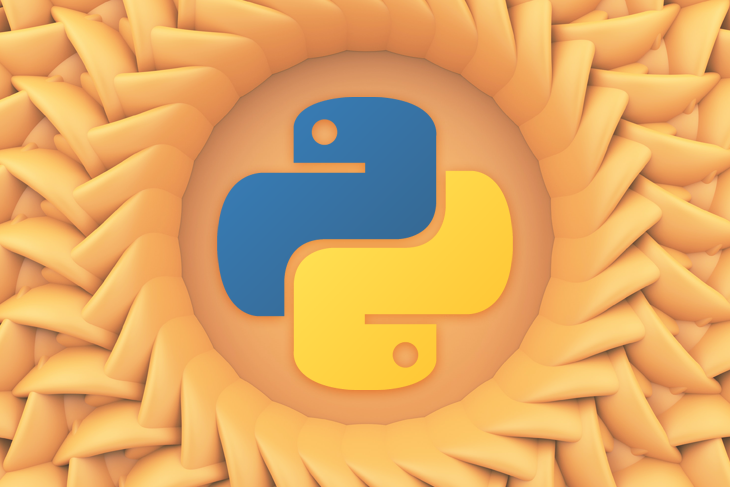## What are loops and when do you use them?

Loops are an essential construct in all programming languages. In a loop structure, the program first checks for a condition. If this condition is true, some piece of code is run. This code will keep on running unless the condition becomes invalid.

For example, look at the following block of pseudo code:

```IF stomach_empty
eat_food()
ENDIF
//check if stomach is empty again.
IF stomach_empty
eat_food()
ENDIF
//check if stomach is still empty,
//....
```

Here, we are checking whether the `stomach_empty` variable is `true`. If this condition is met, the program will execute the `eat_food` method. Furthermore, notice that we are typing the same code multiple times, which means that this breaks the DRY rule of programming.

To mitigate this problem, we can use a loop structure like so:

```WHILE stomach_empty //this code will keep on running if stomach_empty is true
eat_food()
ENDWHILE
```

In this code, we are using a `while` statement. Here, the loop first analyzes whether the `stomach_empty` Boolean is `true`. If this condition is satisfied, the program keeps on running the `eat_food` function until the condition becomes false. We will learn about `while` loops later in this article.

To summarize, developers use loops to run a piece of code multiple times until a certain condition is met. As a result, this saves time and promotes code readability.

### Types of loops

In Python, there are two kinds of loop structures:

• `for`: Iterate a predefined number of times. This is also known as a definite iteration
• `while`: Keep on iterating until the condition is `false`. This is known as an indefinite iteration

## `for` loops

A `for` loop is a type of loop that runs for a preset number of times. It also has the ability to iterate over the items of any sequence, such as a list or a string.

### Syntax

```for i in <collection>:
<loop body>
```

Here, `collection` is a list of objects. The loop variable, `i`, takes on the value of the next element in `collection` each time through the loop. The code within `loop body` keeps on running until `i` reaches the end of the collection.

### Looping with numbers

To demonstrate `for` loops, let’s use a numeric range loop:

```for i in range(10):  # collection of numbers from 0 to 9
print(i)
```

In this piece of code, we used the `range` function to create a collection of numbers from 0 to 9. Later on, we used the `print` function to log out the value of our loop variable, `i`. As a result, this will output the list of numbers ranging from 0 to 9.

The `range(<end>)` method returns an iterable that returns integers starting with 0, up to but not including `<end>` .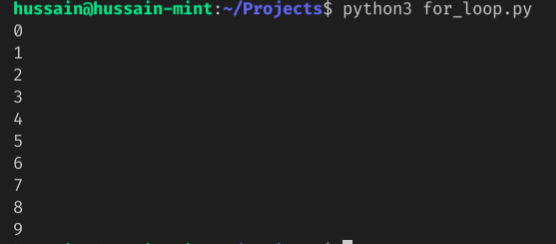We can even use conditional statements in our loops like so:

```for i in range(10):  # numbers from 0-9
if i % 2 == 0:  # is divsible by 2? (even number)
print(i) # then print.
```

This block of code will output all even numbers ranging from 0 to 9.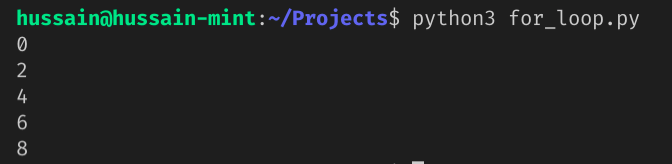### Looping with lists

We can even use a `for` loop to iterate through lists:

```names = ["Bill Gates", "Steve Jobs", "Mark Zuckerberg"] # create our list
for name in names:  # load our list of names and iterate through them
print(name)
```

In the above snippet, we created a list called `names`. Later on, we used the `for` command to iterate through the `names` array and then logged out the contents of this list.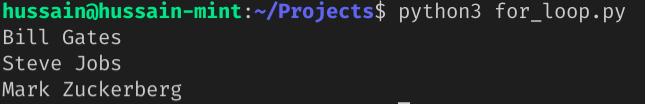The snippet below uses an `if` statement to return all the names that include letter ‘B’:

```names = ["Bill Gates", "Billie Eilish", "Mark Zuckerberg"]  # create our list
for name in names:  # load our list of names and iterate through them
if "B" in name:  # does the name include 'B'?
print(name)
```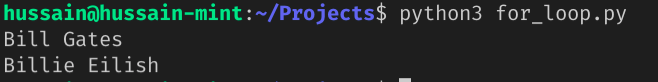## List comprehension

In some cases, you might want to create a new list based off the data of an existing list.
For example, look at the following code:

```names = ["Bill Gates", "Billie Eilish", "Mark Zuckerberg", "Hussain"]
namesWithB = []
for name in names:
if "B" in name:
namesWithB.append(name) # add this element to this array.
print(namesWithB)
```

In this code, we used the `for` command to iterate through the `names` array and then checked whether any element contains the letter `B`. If true, the program appends this corresponding element to the `namesWithB` list.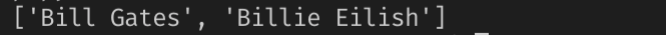Using the power of list comprehension, we can shrink this block of code by a large extent.

### Syntax

```newlist = [<expression> for <loop variable> in <list> (if condition)]
```

Here, `expression` can be a piece of code that returns a value, for example, a method. The elements of `list` will be appended to the `newlist` array if `loop variable` fulfills the `condition`.

### Usage with lists

Let’s rewrite our code that we wrote earlier using list comprehension:

```names = ["Bill Gates", "Billie Eilish", "Mark Zuckerberg", "Hussain"]
namesWithB = [name for name in names if "B" in name]
print(namesWithB)
```

In this code, we iterated through the `names` array. According to our condition, all elements containing the letter `B` will be added to the `namesWithB` list.### Usage with numbers

We can use the `range` method in list comprehension like so:

```numbers = [i for i in range(10)]
print(numbers)
```

Notice that in this case, we don’t have a conditional statement. This means that conditions are optional.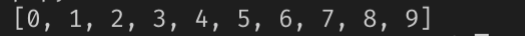This snippet of code will use a condition to get the list of even numbers between 0 and 9: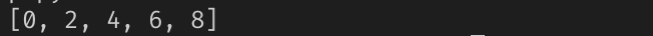## `while` loops

With the `while` loop, we can execute a block of code as long as a `condition` is `true`.

### Syntax

```while <condition>:
<loop body>
```

In a `while` loop, the `condition` is first checked. If it is `true` , the code in `loop body` is executed. This process will repeat until the `condition` becomes `false`.

### Looping with numbers

This piece of code prints out integers between `0` and `9` .

```n = 0
while n < 10: # while n is less than 10,
print(n) # print out the value of n
n += 1 #
```

Here’s what’s happening in this example:

• The initial value of `n` is `0`. The program first checks whether `n` is more than `10`. Since this is `true`, the loop body runs
• Within the loop, we are first printing out the value of `n`. Later on, we are incrementing it by `1`.
• When the body of the loop has finished, program execution evaluates the condition again. Because it is still true, the body executes again.
• This continues until `n` exceeds `10`. At this point, when the expression is tested, it is `false`, and the loop halts.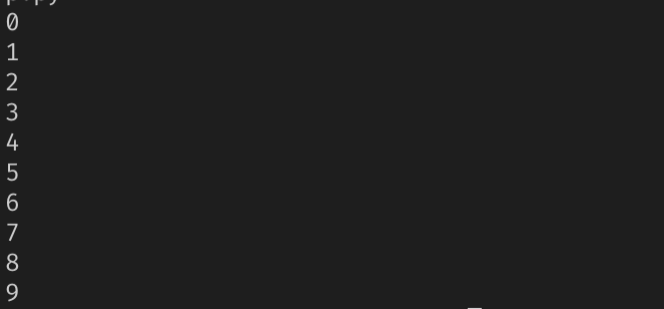### Looping with lists

We can use a `while` block to iterate through lists like so:

```numbers = [0, 5, 10, 6, 3]
length = len(numbers) # get length of array.
n = 0
while n < length: # loop condition
print(numbers[n])
n += 1
```

Here’s a breakdown of this program:

• The `len` function returns the number of elements present in the `numbers` array
• Our `while` statement first checks whether `n` is less than the `length` variable. Since this is true, the program will print out the items in the `numbers` list. In the end, we are incrementing the `n` variable
• When `n` exceeds `length` , the loop halts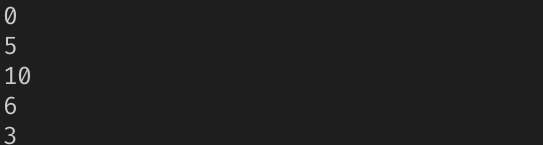## Loop control statements

There are three loop control statements:

• `break`: Terminates the loop if a specific condition is met
• `continue`: Skips one iteration of the loop if a specified condition is met, and continues with the next iteration. The difference between `continue` and `break` is that the `break` keyword will “jump out” of the loop, but `continue` will “jump over” one cycle of the loop
• `pass`: When you don’t want any command or code to execute.

We can use all of these in both `while` and `for` loops.

### 1. `break`

The `break` statement is useful when you want to exit out of the loop if some condition is `true`.
Here is the `break` keyword in action:

```names = ["Bill Gates", "Billie Eilish", "Mark Zuckerberg", "Hussain"]
for name in names:
if name == "Mark Zuckerberg":
print("loop exit here.")
break  # end this loop if condition is true.
print(name)
print("Out of the loop")
```

A few inferences from this code:

• The program first iterates through the `names` array
• During each cycle, Python checks whether the current value of `name` is `Mark Zuckerberg`
• If the above condition is satisfied, the program will tell the user that it has halted the loop
• However, if the condition is `false`, the program will print the value of `name`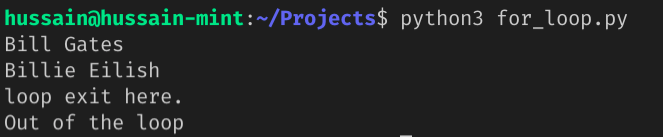### 2. `continue`

The `continue` statement tells Python to skip over the current iteration and move on with the next.
Here is an example:

```names = ["Bill Gates", "Billie Eilish", "Mark Zuckerberg", "Hussain"]
for name in names:
if name == "Mark Zuckerberg":
print("Skipping this iteration.")
continue  # Skip iteration if true.
print(name)
print("Out of the loop")
```

Here is a breakdown of this script:

• Go through the `names` array
• If the app encounters an element that has the value `Mark Zuckerberg`, use the `continue` statement to jump over this iteration
• Otherwise, print out the value of our loop counter, `name`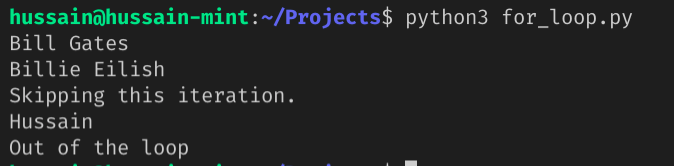### 3. `pass`

Use the `pass` statement if you don’t want any command to run. In other words, `pass` allows you to perform a “null” operation. This can be crucial in places where your code will go but has not been written yet.

Here is a simple example of the `pass` keyword:

```names = ["Bill Gates", "Billie Eilish", "Mark Zuckerberg", "Hussain"]
for name in names:
if name == "Mark Zuckerberg":
print("Just passing by...")
pass  # Move on with this iteration
print(name)
print("Out of the loop")
```

This will be the output: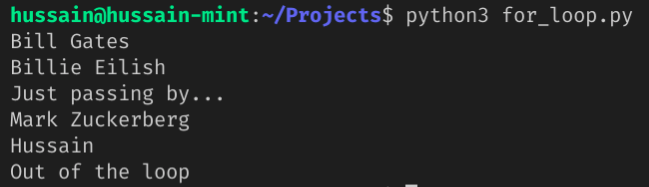### 4. The `else` clause

Python allows us to append `else` statements to our loops as well. The code within the `else` block executes when the loop terminates.
Here is the syntax:

```# for 'for' loops
for i in <collection>:
<loop body>
else:
<code block> # will run when loop halts.
# for 'while' loops
while <condition>:
<loop body>
else:
<code block> # will run when loop halts
```

Here, one might think, “Why not put the code in `code` `block` immediately after the loop? Won’t it accomplish the same thing?”

There is a minor difference. Without `else`, `code block` will run after the loop terminates, no matter what.

However, with the `else` statement, `code block` will not run if the loop terminates via the `break` keyword.

Here is an example to properly understand its purpose:

```names = ["Bill Gates", "Billie Eilish", "Mark Zuckerberg", "Hussain"]
print("Else won't run here.")
for name in names:
if name == "Mark Zuckerberg":
print("Loop halted due to break")
break  # Halt this loop
print(name)
else: # this won't work because 'break' was used.
print("Loop has finished")

print(" \n Else statement will run here:")
for name in names:
print(name)
else: # will work because of no 'break' statement
print("second Loop has finished")
```

This will be the output: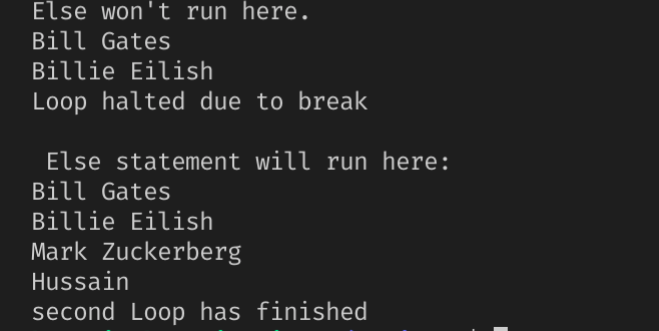## Conclusion

In this article, you learned how to use the `for` and `while` loop in Python programming. Moreover, you also learned about the fundamentals of list comprehension and loop altering statements. These are crucial concepts that help you in increasing your Python skills.

Thank you so much for reading!

# Get set up with LogRocket's modern error tracking in minutes:

1. Visit https://logrocket.com/signup/ to get an app ID
2. Install LogRocket via npm or script tag. `LogRocket.init()` must be called client-side, not server-side
3. `\$ npm i --save logrocket // Code:import LogRocket from 'logrocket'; LogRocket.init('app/id');`
`Add to your HTML:<script src="https://cdn.lr-ingest.com/LogRocket.min.js"></script><script>window.LogRocket && window.LogRocket.init('app/id');</script>`
4. (Optional) Install plugins for deeper integrations with your stack:
• Redux middleware
• NgRx middleware
• Vuex pluginHussain Arif Hussain is a CS student in Pakistan whose biggest interest is learning and teaching programming to make the world a better place.